# Mean State

Period Mean (original grids) [W/m2]
Model Period Mean (intersection) [W/m2]
Model Period Mean (complement) [W/m2]
Benchmark Period Mean (intersection) [W/m2]
Benchmark Period Mean (complement) [W/m2]
Bias [W/m2]
RMSE [W/m2]
Phase Shift [months]
Bias Score 
RMSE Score 
Seasonal Cycle Score 
Spatial Distribution Score 
Interannual Variability Score 
Overall Score 
Benchmark [-] -56.1
CLM5PHSOFF [-] -65.5 -65.7 -56.5 -49.8 -9.31 16.9 1.83 0.494 0.405 0.737 0.972 0.749 0.627
CLM5PHSON [-] -65.8 -65.8 -56.5 -49.8 -9.20 16.5 1.82 0.506 0.413 0.738 0.979 0.735 0.631
Period Mean (original grids) [W/m2]
Model Period Mean (intersection) [W/m2]
Model Period Mean (complement) [W/m2]
Benchmark Period Mean (intersection) [W/m2]
Benchmark Period Mean (complement) [W/m2]
Bias [W/m2]
RMSE [W/m2]
Phase Shift [months]
Bias Score 
RMSE Score 
Seasonal Cycle Score 
Spatial Distribution Score 
Interannual Variability Score 
Overall Score 
Benchmark [-] -75.5
CLM5PHSOFF [-] -97.7 -98.3 -75.8 -54.3 -22.3 27.3 0.310 0.452 0.568 0.969 0.906 0.731 0.699
CLM5PHSON [-] -97.5 -98.2 -75.8 -54.3 -22.0 27.2 0.353 0.457 0.567 0.965 0.952 0.702 0.702
Period Mean (original grids) [W/m2]
Model Period Mean (intersection) [W/m2]
Model Period Mean (complement) [W/m2]
Benchmark Period Mean (intersection) [W/m2]
Benchmark Period Mean (complement) [W/m2]
Bias [W/m2]
RMSE [W/m2]
Phase Shift [months]
Bias Score 
RMSE Score 
Seasonal Cycle Score 
Spatial Distribution Score 
Interannual Variability Score 
Overall Score 
Benchmark [-] -76.3
CLM5PHSOFF [-] -91.1 -91.5 -77.0 -61.8 -14.5 21.7 1.05 0.485 0.516 0.870 0.995 0.785 0.695
CLM5PHSON [-] -91.0 -91.1 -77.0 -61.8 -12.1 20.7 1.11 0.531 0.501 0.862 0.995 0.760 0.692
Period Mean (original grids) [W/m2]
Model Period Mean (intersection) [W/m2]
Model Period Mean (complement) [W/m2]
Benchmark Period Mean (intersection) [W/m2]
Benchmark Period Mean (complement) [W/m2]
Bias [W/m2]
RMSE [W/m2]
Phase Shift [months]
Bias Score 
RMSE Score 
Seasonal Cycle Score 
Spatial Distribution Score 
Interannual Variability Score 
Overall Score 
Benchmark [-] -36.2
CLM5PHSOFF [-] -41.0 -41.0 -35.9 -39.8 -5.08 18.9 1.69 0.645 0.360 0.779 0.949 0.877 0.662
CLM5PHSON [-] -40.2 -40.3 -35.9 -39.8 -4.97 18.6 1.64 0.655 0.365 0.788 0.945 0.879 0.666
Period Mean (original grids) [W/m2]
Model Period Mean (intersection) [W/m2]
Model Period Mean (complement) [W/m2]
Benchmark Period Mean (intersection) [W/m2]
Benchmark Period Mean (complement) [W/m2]
Bias [W/m2]
RMSE [W/m2]
Phase Shift [months]
Bias Score 
RMSE Score 
Seasonal Cycle Score 
Spatial Distribution Score 
Interannual Variability Score 
Overall Score 
Benchmark [-] -46.4
CLM5PHSOFF [-] -46.0 -46.0 -45.1 -51.0 -1.04 12.5 2.00 0.383 0.358 0.707 0.959 0.735 0.583
CLM5PHSON [-] -47.5 -47.5 -45.1 -51.0 -1.79 12.4 1.98 0.386 0.358 0.707 0.940 0.732 0.580
Period Mean (original grids) [W/m2]
Model Period Mean (intersection) [W/m2]
Model Period Mean (complement) [W/m2]
Benchmark Period Mean (intersection) [W/m2]
Benchmark Period Mean (complement) [W/m2]
Bias [W/m2]
RMSE [W/m2]
Phase Shift [months]
Bias Score 
RMSE Score 
Seasonal Cycle Score 
Spatial Distribution Score 
Interannual Variability Score 
Overall Score 
Benchmark [-] -44.2
CLM5PHSOFF [-] -53.5 -53.5 -44.1 -53.1 -9.50 16.3 1.11 0.536 0.449 0.872 0.882 0.889 0.680
CLM5PHSON [-] -52.2 -52.2 -44.1 -53.1 -8.12 15.1 1.02 0.577 0.464 0.883 0.849 0.886 0.687
Period Mean (original grids) [W/m2]
Model Period Mean (intersection) [W/m2]
Model Period Mean (complement) [W/m2]
Benchmark Period Mean (intersection) [W/m2]
Benchmark Period Mean (complement) [W/m2]
Bias [W/m2]
RMSE [W/m2]
Phase Shift [months]
Bias Score 
RMSE Score 
Seasonal Cycle Score 
Spatial Distribution Score 
Interannual Variability Score 
Overall Score 
Benchmark [-] -66.8
CLM5PHSOFF [-] -73.2 -73.0 -67.0 -58.2 -5.92 16.9 1.16 0.642 0.512 0.848 0.983 0.823 0.720
CLM5PHSON [-] -72.9 -72.6 -67.0 -58.2 -4.65 16.5 1.10 0.665 0.507 0.857 0.989 0.826 0.725
Period Mean (original grids) [W/m2]
Model Period Mean (intersection) [W/m2]
Model Period Mean (complement) [W/m2]
Benchmark Period Mean (intersection) [W/m2]
Benchmark Period Mean (complement) [W/m2]
Bias [W/m2]
RMSE [W/m2]
Phase Shift [months]
Bias Score 
RMSE Score 
Seasonal Cycle Score 
Spatial Distribution Score 
Interannual Variability Score 
Overall Score 
Benchmark [-] -60.6
CLM5PHSOFF [-] -78.0 -78.5 -61.3 -51.8 -17.3 23.7 1.05 0.484 0.537 0.860 0.988 0.750 0.693
CLM5PHSON [-] -79.0 -79.2 -61.3 -51.8 -15.1 22.5 1.07 0.519 0.525 0.856 0.988 0.738 0.692
Period Mean (original grids) [W/m2]
Model Period Mean (intersection) [W/m2]
Model Period Mean (complement) [W/m2]
Benchmark Period Mean (intersection) [W/m2]
Benchmark Period Mean (complement) [W/m2]
Bias [W/m2]
RMSE [W/m2]
Phase Shift [months]
Bias Score 
RMSE Score 
Seasonal Cycle Score 
Spatial Distribution Score 
Interannual Variability Score 
Overall Score 
Benchmark [-] -32.3
CLM5PHSOFF [-] -40.8 -40.9 -32.2 -34.9 -8.71 18.9 2.12 0.558 0.361 0.710 0.924 0.901 0.636
CLM5PHSON [-] -40.5 -40.5 -32.2 -34.9 -8.31 18.5 2.10 0.572 0.366 0.715 0.938 0.901 0.643
Period Mean (original grids) [W/m2]
Model Period Mean (intersection) [W/m2]
Model Period Mean (complement) [W/m2]
Benchmark Period Mean (intersection) [W/m2]
Benchmark Period Mean (complement) [W/m2]
Bias [W/m2]
RMSE [W/m2]
Phase Shift [months]
Bias Score 
RMSE Score 
Seasonal Cycle Score 
Spatial Distribution Score 
Interannual Variability Score 
Overall Score 
Benchmark [-] -88.6
CLM5PHSOFF [-] -95.8 -95.9 -90.4 -66.1 -5.55 15.0 0.799 0.662 0.531 0.894 0.992 0.850 0.743
CLM5PHSON [-] -98.3 -98.4 -90.4 -66.1 -4.59 15.0 0.857 0.668 0.527 0.886 0.985 0.836 0.738
Period Mean (original grids) [W/m2]
Model Period Mean (intersection) [W/m2]
Model Period Mean (complement) [W/m2]
Benchmark Period Mean (intersection) [W/m2]
Benchmark Period Mean (complement) [W/m2]
Bias [W/m2]
RMSE [W/m2]
Phase Shift [months]
Bias Score 
RMSE Score 
Seasonal Cycle Score 
Spatial Distribution Score 
Interannual Variability Score 
Overall Score 
Benchmark [-] -54.5
CLM5PHSOFF [-] -60.4 -60.3 -54.0 -59.9 -6.30 14.2 1.51 0.618 0.458 0.784 0.990 0.884 0.699
CLM5PHSON [-] -58.1 -58.1 -54.0 -59.9 -4.66 13.9 1.59 0.660 0.446 0.770 0.976 0.885 0.697
Period Mean (original grids) [W/m2]
Model Period Mean (intersection) [W/m2]
Model Period Mean (complement) [W/m2]
Benchmark Period Mean (intersection) [W/m2]
Benchmark Period Mean (complement) [W/m2]
Bias [W/m2]
RMSE [W/m2]
Phase Shift [months]
Bias Score 
RMSE Score 
Seasonal Cycle Score 
Spatial Distribution Score 
Interannual Variability Score 
Overall Score 
Benchmark [-] -53.4
CLM5PHSOFF [-] -71.3 -71.3 -61.2 -49.6 -10.1 19.0 1.31 0.541 0.469 0.818 0.979 0.779 0.676
CLM5PHSON [-] -71.7 -71.7 -61.2 -49.6 -9.06 18.5 1.31 0.563 0.469 0.818 0.976 0.773 0.678
Period Mean (original grids) [W/m2]
Model Period Mean (intersection) [W/m2]
Model Period Mean (complement) [W/m2]
Benchmark Period Mean (intersection) [W/m2]
Benchmark Period Mean (complement) [W/m2]
Bias [W/m2]
RMSE [W/m2]
Phase Shift [months]
Bias Score 
RMSE Score 
Seasonal Cycle Score 
Spatial Distribution Score 
Interannual Variability Score 
Overall Score 
Benchmark [-] -100.
CLM5PHSOFF [-] -108. -108. -101. -73.0 -6.63 16.9 0.849 0.626 0.513 0.880 0.971 0.801 0.717
CLM5PHSON [-] -108. -108. -101. -73.0 -6.02 16.9 0.860 0.631 0.511 0.879 0.984 0.802 0.720
Period Mean (original grids) [W/m2]
Model Period Mean (intersection) [W/m2]
Model Period Mean (complement) [W/m2]
Benchmark Period Mean (intersection) [W/m2]
Benchmark Period Mean (complement) [W/m2]
Bias [W/m2]
RMSE [W/m2]
Phase Shift [months]
Bias Score 
RMSE Score 
Seasonal Cycle Score 
Spatial Distribution Score 
Interannual Variability Score 
Overall Score 
Benchmark [-] -33.5
CLM5PHSOFF [-] -44.0 -44.0 -33.5 -34.8 -10.6 19.3 2.12 0.546 0.391 0.680 0.990 0.837 0.639
CLM5PHSON [-] -43.3 -43.3 -33.5 -34.8 -10.2 19.1 2.13 0.557 0.390 0.678 0.990 0.836 0.640
Period Mean (original grids) [W/m2]
Model Period Mean (intersection) [W/m2]
Model Period Mean (complement) [W/m2]
Benchmark Period Mean (intersection) [W/m2]
Benchmark Period Mean (complement) [W/m2]
Bias [W/m2]
RMSE [W/m2]
Phase Shift [months]
Bias Score 
RMSE Score 
Seasonal Cycle Score 
Spatial Distribution Score 
Interannual Variability Score 
Overall Score 
Benchmark [-] -66.0
CLM5PHSOFF [-] -82.5 -82.7 -67.8 -57.2 -14.9 22.1 2.10 0.405 0.439 0.649 0.960 0.812 0.617
CLM5PHSON [-] -84.7 -84.6 -67.8 -57.2 -13.3 20.9 2.06 0.435 0.442 0.654 0.967 0.805 0.624
Period Mean (original grids) [W/m2]
Model Period Mean (intersection) [W/m2]
Model Period Mean (complement) [W/m2]
Benchmark Period Mean (intersection) [W/m2]
Benchmark Period Mean (complement) [W/m2]
Bias [W/m2]
RMSE [W/m2]
Phase Shift [months]
Bias Score 
RMSE Score 
Seasonal Cycle Score 
Spatial Distribution Score 
Interannual Variability Score 
Overall Score 
Benchmark [-] -51.6
CLM5PHSOFF [-] -65.2 -65.2 -51.4 -56.8 -13.8 17.8 1.91 0.339 0.415 0.683 0.842 0.899 0.599
CLM5PHSON [-] -62.9 -62.7 -51.4 -56.8 -11.3 15.7 1.88 0.416 0.424 0.697 0.825 0.901 0.614
Period Mean (original grids) [W/m2]
Model Period Mean (intersection) [W/m2]
Model Period Mean (complement) [W/m2]
Benchmark Period Mean (intersection) [W/m2]
Benchmark Period Mean (complement) [W/m2]
Bias [W/m2]
RMSE [W/m2]
Phase Shift [months]
Bias Score 
RMSE Score 
Seasonal Cycle Score 
Spatial Distribution Score 
Interannual Variability Score 
Overall Score 
Benchmark [-] -46.1
CLM5PHSOFF [-] -54.6 -54.6 -46.1 -44.3 -8.44 14.4 0.785 0.596 0.502 0.913 0.933 0.856 0.717
CLM5PHSON [-] -53.2 -53.2 -46.1 -44.3 -6.84 13.5 0.817 0.652 0.503 0.907 0.890 0.862 0.720
Period Mean (original grids) [W/m2]
Model Period Mean (intersection) [W/m2]
Model Period Mean (complement) [W/m2]
Benchmark Period Mean (intersection) [W/m2]
Benchmark Period Mean (complement) [W/m2]
Bias [W/m2]
RMSE [W/m2]
Phase Shift [months]
Bias Score 
RMSE Score 
Seasonal Cycle Score 
Spatial Distribution Score 
Interannual Variability Score 
Overall Score 
Benchmark [-] -78.8
CLM5PHSOFF [-] -89.3 -89.1 -79.2 -53.1 -10.0 17.8 1.20 0.619 0.547 0.832 0.992 0.862 0.733
CLM5PHSON [-] -88.1 -87.9 -79.2 -53.1 -8.35 17.1 1.20 0.650 0.545 0.832 0.994 0.865 0.739
Period Mean (original grids) [W/m2]
Model Period Mean (intersection) [W/m2]
Model Period Mean (complement) [W/m2]
Benchmark Period Mean (intersection) [W/m2]
Benchmark Period Mean (complement) [W/m2]
Bias [W/m2]
RMSE [W/m2]
Phase Shift [months]
Bias Score 
RMSE Score 
Seasonal Cycle Score 
Spatial Distribution Score 
Interannual Variability Score 
Overall Score 
Benchmark [-] -39.8
CLM5PHSOFF [-] -52.3 -52.1 -39.6 -48.2 -12.6 17.6 1.67 0.327 0.421 0.764 0.900 0.700 0.589
CLM5PHSON [-] -51.8 -51.6 -39.6 -48.2 -12.1 17.0 1.70 0.326 0.430 0.760 0.965 0.682 0.599
Period Mean (original grids) [W/m2]
Model Period Mean (intersection) [W/m2]
Model Period Mean (complement) [W/m2]
Benchmark Period Mean (intersection) [W/m2]
Benchmark Period Mean (complement) [W/m2]
Bias [W/m2]
RMSE [W/m2]
Phase Shift [months]
Bias Score 
RMSE Score 
Seasonal Cycle Score 
Spatial Distribution Score 
Interannual Variability Score 
Overall Score 
Benchmark [-] -63.6
CLM5PHSOFF [-] -73.8 -74.2 -63.7 -59.9 -10.5 17.2 1.25 0.556 0.486 0.836 0.983 0.798 0.691
CLM5PHSON [-] -72.4 -72.8 -63.7 -59.9 -8.42 16.1 1.27 0.619 0.485 0.833 0.982 0.768 0.695
Period Mean (original grids) [W/m2]
Model Period Mean (intersection) [W/m2]
Model Period Mean (complement) [W/m2]
Benchmark Period Mean (intersection) [W/m2]
Benchmark Period Mean (complement) [W/m2]
Bias [W/m2]
RMSE [W/m2]
Phase Shift [months]
Bias Score 
RMSE Score 
Seasonal Cycle Score 
Spatial Distribution Score 
Interannual Variability Score 
Overall Score 
Benchmark [-] -80.2
CLM5PHSOFF [-] -84.9 -84.6 -83.6 -50.6 -0.767 21.7 1.22 0.543 0.456 0.839 0.930 0.773 0.666
CLM5PHSON [-] -85.8 -85.5 -83.6 -50.6 1.06 21.5 1.21 0.556 0.455 0.841 0.917 0.776 0.667

# Temporally integrated period mean

BENCHMARK MEAN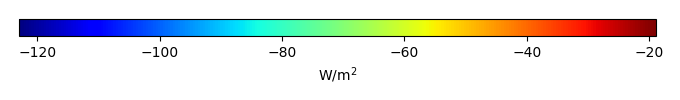MODEL MEANBIAS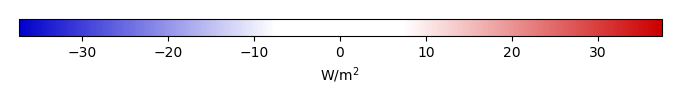BIAS SCORERMSE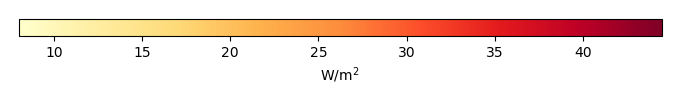RMSE SCOREBENCHMARK INTERANNUAL VARIABILITY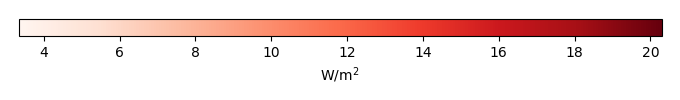MODEL INTERANNUAL VARIABILITYINTERANNUAL VARIABILITY SCOREBENCHMARK MAX MONTHMODEL MAX MONTHDIFFERENCE IN MAX MONTH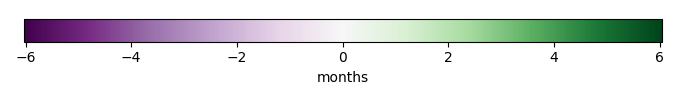SEASONAL CYCLE SCORESPATIAL TAYLOR DIAGRAMMODEL COLORS# Spatially integrated regional mean

MODEL COLORSREGIONAL MEANANNUAL CYCLEMONTHLY ANOMALYANNUAL CYCLE# All Models

BenchmarkCLM5PHSOFFCLM5PHSON# Data Information

creation_date: Thu May 8 23:08:21 PDT 2014

source_file: This product is generated from monthly 1 degree CERES EBAF Radiation observations